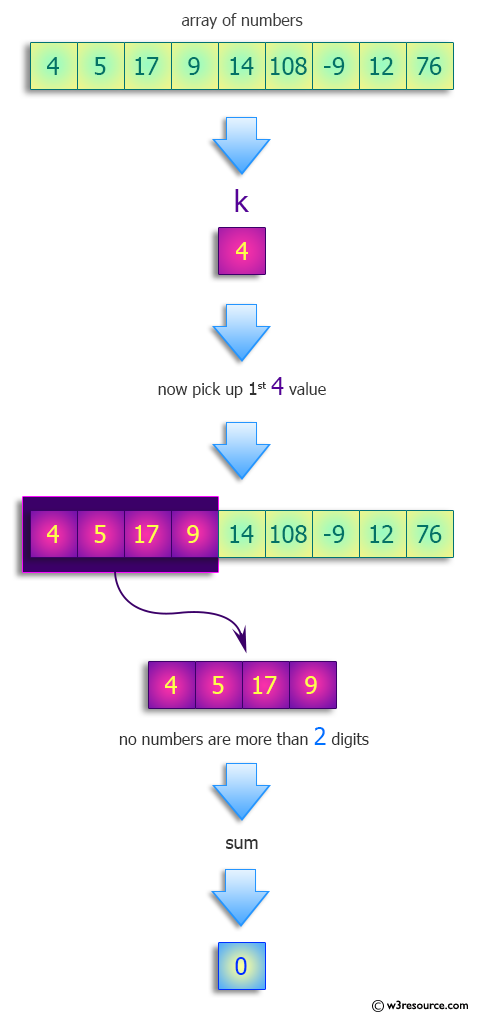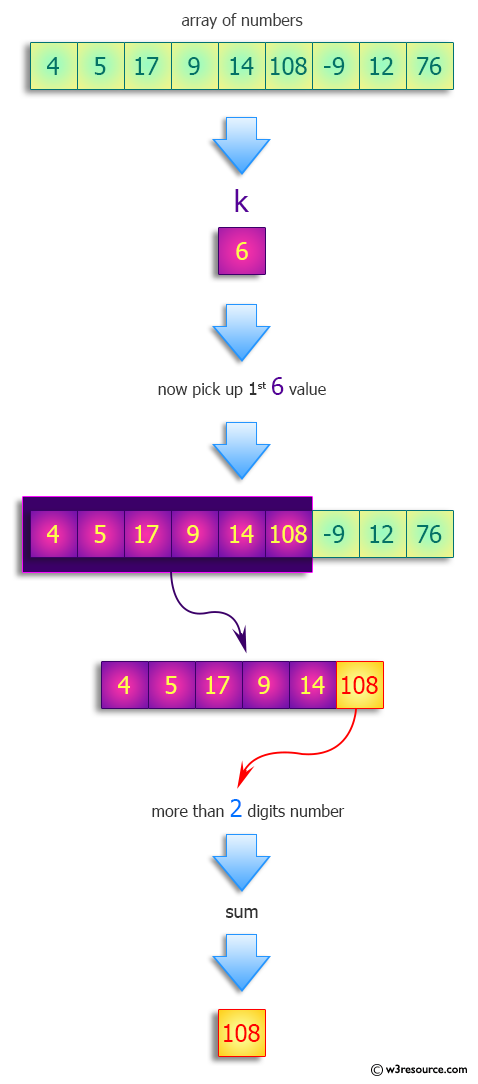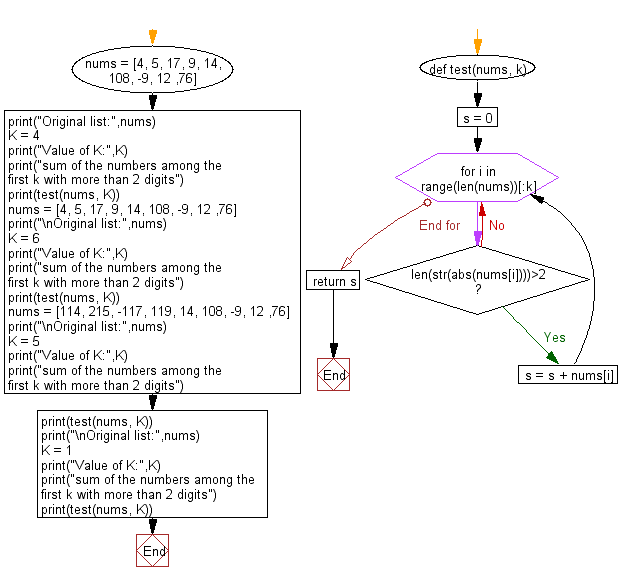﻿ Python: Find the sum of the numbers among the first k with more than 2 digits - w3resource# Python: Find the sum of the numbers among the first k with more than 2 digits

## Python Programming Puzzles: Exercise-34 with Solution

Write a Python program to find the sum of the numbers of a given list among the first k with more than 2 digits.

```Input:
[4, 5, 17, 9, 14, 108, -9, 12, 76]
Value of K: 4
Output:
0

Input:
[4, 5, 17, 9, 14, 108, -9, 12, 76]
Value of K: 6
Output:
108

Input:
[114, 215, -117, 119, 14, 108, -9, 12, 76]
Value of K: 5
Output:
331

Input:
[114, 215, -117, 119, 14, 108, -9, 12, 76]
Value of K: 1
Output:
114
```

Pictorial Presentation:Sample Solution-1:

Python Code:

``````#License: https://bit.ly/3oLErEI

def test(nums, k):
s = 0
for i in range(len(nums))[:k]:
if len(str(abs(nums[i])))>2:
s = s + nums[i]
return s

nums = [4, 5, 17, 9, 14, 108, -9, 12 ,76]
print("Original list:",nums)
K = 4
print("Value of K:",K)
print("sum of the numbers among the first k with more than 2 digits")
print(test(nums, K))
nums = [4, 5, 17, 9, 14, 108, -9, 12 ,76]
print("\nOriginal list:",nums)
K = 6
print("Value of K:",K)
print("sum of the numbers among the first k with more than 2 digits")
print(test(nums, K))
nums = [114, 215, -117, 119, 14, 108, -9, 12 ,76]
print("\nOriginal list:",nums)
K = 5
print("Value of K:",K)
print("sum of the numbers among the first k with more than 2 digits")
print(test(nums, K))
print("\nOriginal list:",nums)
K = 1
print("Value of K:",K)
print("sum of the numbers among the first k with more than 2 digits")
print(test(nums, K))
``````

Sample Output:

```Original list: [4, 5, 17, 9, 14, 108, -9, 12, 76]
Value of K: 4
sum of the numbers among the first k with more than 2 digits
0

Original list: [4, 5, 17, 9, 14, 108, -9, 12, 76]
Value of K: 6
sum of the numbers among the first k with more than 2 digits
108

Original list: [114, 215, -117, 119, 14, 108, -9, 12, 76]
Value of K: 5
sum of the numbers among the first k with more than 2 digits
331

Original list: [114, 215, -117, 119, 14, 108, -9, 12, 76]
Value of K: 1
sum of the numbers among the first k with more than 2 digits
114
```

Flowchart:## Visualize Python code execution:

The following tool visualize what the computer is doing step-by-step as it executes the said program:

Sample Solution-2:

Python Code:

``````#License: https://bit.ly/3oLErEI

def test(nums, k):
return sum(n for n in nums[:k] if len(str(abs(n))) > 2)

nums = [4, 5, 17, 9, 14, 108, -9, 12 ,76]
print("Original list:",nums)
K = 4
print("Value of K:",K)
print("sum of the numbers among the first k with more than 2 digits")
print(test(nums, K))
nums = [4, 5, 17, 9, 14, 108, -9, 12 ,76]
print("\nOriginal list:",nums)
K = 6
print("Value of K:",K)
print("sum of the numbers among the first k with more than 2 digits")
print(test(nums, K))
nums = [114, 215, -117, 119, 14, 108, -9, 12 ,76]
print("\nOriginal list:",nums)
K = 5
print("Value of K:",K)
print("sum of the numbers among the first k with more than 2 digits")
print(test(nums, K))
print("\nOriginal list:",nums)
K = 1
print("Value of K:",K)
print("sum of the numbers among the first k with more than 2 digits")
print(test(nums, K))
``````

Sample Output:

```Original list: [4, 5, 17, 9, 14, 108, -9, 12, 76]
Value of K: 4
sum of the numbers among the first k with more than 2 digits
0

Original list: [4, 5, 17, 9, 14, 108, -9, 12, 76]
Value of K: 6
sum of the numbers among the first k with more than 2 digits
108

Original list: [114, 215, -117, 119, 14, 108, -9, 12, 76]
Value of K: 5
sum of the numbers among the first k with more than 2 digits
331

Original list: [114, 215, -117, 119, 14, 108, -9, 12, 76]
Value of K: 1
sum of the numbers among the first k with more than 2 digits
114
```

Flowchart:## Visualize Python code execution:

The following tool visualize what the computer is doing step-by-step as it executes the said program:

Python Code Editor :

Have another way to solve this solution? Contribute your code (and comments) through Disqus.

What is the difficulty level of this exercise?

Test your Programming skills with w3resource's quiz.

﻿

## Python: Tips of the Day

Clamps num within the inclusive range specified by the boundary values x and y:

Example:

```def tips_clamp_num(num,x,y):
return max(min(num, max(x, y)), min(x, y))
print(tips_clamp_num(2, 4, 6))
print(tips_clamp_num(1, -1, -6))
```

Output:

```4
-1
```